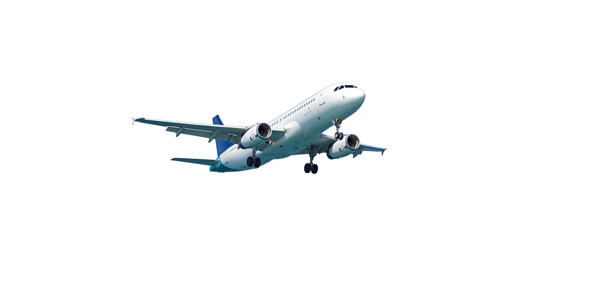# Aircraft Facts And Questions! Trivia Test! Quiz

20 Questions | Total Attempts: 396SettingsCreate your own QuizWhen it comes to the fastest means of transport in the world today air travel is undoubtedly the answer. Do you know how to operate an aircraft and maintain it in tiptop position? This trivia test quiz is made up of facts and questions on things about aircrafts that only the smartest would know. How about you check it out and see if you are among this group. All the best!

• 1.
Bernoulli's Principle states:
• A.

For every action there is an equal and opposite reaction.

• B.

Force equals mass times acceleration.

• C.

As the velocity of a fluid increases, the pressure exerted by the fluid decreases.

• D.

None of the above is correct.

• 2.
The hot air balloon that made the first manned ascent was designed by
• A.

The Montgolfier brothers.

• B.

The Wright brothers.

• C.

Marquis Francois d'Arlandes and Pilatre d'Rozier.

• D.

Chuck Yeager

• 3.
Which engine does not require atmospheric air?
• A.

Reciprocating Engine

• B.

Jet

• C.

V-Inline Engine

• D.

Rocket

• 4.
In this picture, what does the number 27 on the runway represent?
• A.

27° true North

• B.

270° magnetic North

• C.

27° magnetic North

• D.

270° true North

• 5.
Hot air is less dense than cold air, which is how balloons filled with hot air can fly.
• A.

True

• B.

False

• 6.
Looking at the diagram, what action does the aircraft do when it rotates around axis # 1.  WORD BANK:  Yaw      Pitch       Roll ** Must be spelled correctly.  If not, it will be counted as WRONG.
• 7.
Looking at the diagram, what action does the aircraft do when it rotates around axis # 2.  WORD BANK:  Yaw      Pitch       Roll ** Must be spelled correctly.  If not, it will be counted as WRONG.
• 8.
Looking at the diagram, what action does the aircraft do when it rotates around axis # 3.  WORD BANK:  Yaw      Pitch       Roll ** Must be spelled correctly.  If not, it will be counted as WRONG.
• 9.
Of all the wing shapes, the best geometry shape for slow flight is:
• A.

Swept Wing - Low Angle

• B.

Swept Wing - High Angle

• C.

Delta Wing

• D.

Straight wing - Constant Chord

• 10.
This reciprocating engine pictured gets its’ name from what math/geometry principle?
• A.

The RADIUS of a circle

• B.

The DIAMETER of a circle

• C.

The ACUTE ANGLE of a triangle

• D.

The PERIMETER of a square

• 11.
There is breathable oxygen in space.
• A.

True

• B.

False

• 12.
What are the three (3) MAIN types of aerospace engines.  (Not the different kinds of each one)
• A.

Reciprocating

• B.

Turboprop

• C.

• D.

Turbojet

• E.

Scramjet

• F.

V-Inline

• G.

Jet (or Turbine Engine)

• H.

Turbofan

• I.

Plasma Drive

• J.

Rocket

• K.

Horizontally-Opposed

• 13.
Air, while made up of different gasses, has the movement properties of a
• A.

Paper

• B.

Solid

• C.

Fluid

• D.

Organism

• E.

All of the Above

• 14.
Of all the wing shapes, the best geometry shape for fast flight is:
• A.

Swept Wing - Low Angle

• B.

Swept Wing - High Angle

• C.

Delta Wing

• D.

Straight wing - Constant Chord

• 15.
Which of the Four Forces of Flight is an aerodynamic force created by the shape of the airfoil?
• A.

Lift

• B.

Weight

• C.

Thrust

• D.

Drag

• 16.
Which of the Four Forces of Flight is not constant and tries to pull the aircraft to the earth?
• A.

Lift

• B.

Weight

• C.

Thrust

• D.

Drag

• 17.
Which of the Four Forces of Flight pushes / pulls the aircraft through the air?
• A.

Lift

• B.

Weight

• C.

Thrust

• D.

Drag

• 18.
Which of the Four Forces of Flight tries to pull the aircraft backward and slows the aircraft through the air?
• A.

Lift

• B.

Weight

• C.

Thrust

• D.

Drag

Related TopicsBack to top
×

Wait!
Here's an interesting quiz for you.